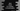# Java program to check if a number is Automorphic or not## Java program to check if a number is Automorphic or not:

In this post, we will learn how to check if a user given number is Automorphic or not in Java. This program will ask the user to enter a number, it will check if that number is Automorphic and print one message based on that.

### Automorphic number:

A number is called an Automorphic number if we find the square of a number, the number will ends in this value.

For example, 25 is an automorphic number because, if we find the square of 25, it is 625 and 25 ends in 625.

But, 10 is not a automorphic number because its square value 100 is ending with 10.

Similarly, 6 is an automorphic number because its square value 36 ends with 6.

### Algorithm to check if a number is automorphic or not:

We can check if a number is automorphic or not by using the following steps:

• Take the number as input from the user.
• Find the square value of the number.
• Keep comparing the last digits of both the numbers.

• If the last digits of both the numbers are equal, remove that digit from both.
• If the last digits of both the numbers are not equal, return false.
• Keep comparing the last digits until all digits are compared for the original number. If all digits are compared and found in the square value, return true as this is an automorphic number.

## Java program:

Below is the complete Java program:

``````import java.util.Scanner;

class Main {
static boolean isAutomorphic(int n) {
int squareN = n * n;
int lastDigitN, lastDigitSquare;

while (n > 0) {
lastDigitN = n % 10;
lastDigitSquare = squareN % 10;

if (lastDigitN != lastDigitSquare) {
return false;
}

n /= 10;
squareN /= 10;
}

return true;
}

public static void main(String[] args) {
Scanner sc = new Scanner(System.in);
int n;

System.out.println("Enter a number: ");
n = sc.nextInt();

if (isAutomorphic(n)) {
System.out.println(n + " is an Automorphic number");
} else {
System.out.println(n + " is not an Automorphic number");
}
}
}``````

### Explanation:

Here,

• isAutomorphic method is used to check if a number is an automorphic number or not. It returns one boolean value based on the provided number.

• squareN is an integer variable initialized as the square of the given number.
• lastDigitN is an integer variable to hold the last digit of the number and lastDigitSquare is to hold the last digit of the number square.
• The while loop will run until the value of n is greater than 0.
• Inside the loop, we are finding the last digit of the number and of the square of the number.
• If both values are not equal, return false, i.e. it is not an automorphic number.
• Remove the last digits of both numbers. We are dividing the numbers by 10 and asssigning that value to the number. This will remove the last digit. If we remove the last digits, the next iteration of the loop will pick the next digits.
• Once the loop ends, i.e. all end digits of the square value matches with the original number. Return true.
• Inside main, we are asking the user to enter a number.
• By using a Scanner object, we are reading this number and this number is stored in n.
• The if-else block is checking if that number is automorphic or not by using the isAutomorphic. Based on the result of isAutomorphic, it prints a message to the user.

### Sample output:

If you run the above program, it will print output as like below:

``````Enter a number:
100
100 is not an Automorphic number

Enter a number:
76
76 is an Automorphic number``````

It printed 76 is an Automorphic number because the square of 76, 5776 holds 76 at the end.